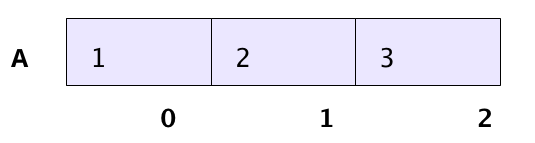Code has been added to clipboard!

# C++ Array: How to Use Arrays

Published Sep 3, 2019
Updated Sep 27, 2019

#### Web Development Course:

TL;DR – C++ has four different data types – int, float, char, and string data. C++ array allows you to have a collection elements in one of these data types.

## What is a C++ Array?

An array is a collection of homogenous data or variables. This means you can store a lot of typical data under the same name with an array. C++ arrays are often used to organize and track data.

An array contains elements that store individual data. The name of the array represents individual data within the elements. The element location is pointed out by an index number which is counted from left to right, starting from zero. Both the array name and the index number is used to access the data:A is the name of the array which consists of three elements. The first element's index is 0, the second element's index is 1, and the third element's index is 2.

Note: an array that contains other arrays is called a multidimensional array.

## C++ Array Syntax

Let’s look at how arrays work by breaking down the syntax.

### Creating an Array

To create an array, you should state its name, data type, and the number of designated elements within square brackets:

• The `type` can be int, float, char, or string data.
• The `array_name` corresponds to the name of the array you’re about to create.
• The `array_size` must be bigger than zero.
Example
``type array_name[array_size];``

### Defining Array Elements

After you create an array, you can define the value of each element by mentioning a previously created array and adding the number of the values after the equal sign (`=`). The value of each element is separated with a comma:

Example
``type array_name[array_size] = { number_of_value,..,..,.. }``

Let's review all the syntax elements to get a better understanding:

• The `array_name` is the name of the array you’re about to input the value to.
• The `number_of_value` corresponds to the individual data’s value you stored within the array’s elements.
• The `number_of_value` within the curly brackets should be less than the `array_size` within the square brackets.

You can also add value to each element individually by defining the element’s index within square brackets and typing the value after an equal sign:

Example
``array_name[index] = number_of_value;``

Note: keep in mind that the value should not be greater than the number of total elements within an array.

### Accessing Array Elements

You can access the value of an array by specifying the array name and the element’s index within square brackets:

Example
``array_name[index];``

The `array_name` is the name of the array you’re about to access, and the `index` corresponds to the location of the elements. You can use the code for both retrieving and storing data within the element.

## Most Common C++ Array Application

Let’s say you want to keep track of a soccer team’s score for five consecutive matches. You have to create five elements of an integer array.

To create an array named soccer_score, which has five integer elements, the code would look like this:

Example
``int soccer_score;``

Start creating you project by using the array and inputting the code. You can directly assign particular values for the array’s elements.

For example, if the team scored 3 points for the first and second matches, then scored 2 for the third match and got 4 points for the last two matches, you will have `{3,3,2,4,4}` as the values for the array:

Example
``int soccer_score = { 3, 3, 2, 4, 4 };``

You can also input the values one by one, like this (although the above method is a time-saver):

Example
``````soccer_score = 3;
soccer_score = 3;
soccer_score = 2;
soccer_score = 4;
soccer_score = 4;``````

After adding values to the elements, we can check a particular element’s value by using the `cout` function. Then, specify the array’s name and elements within square brackets between an insertion operator and close it with `endl`.

For instance, to see the third element’s value, you should point out the index of `2`:

Example
``cout << soccer_score << endl;``

Here’s the output:

Example
``````#include <iostream>

using namespace std;

int main() {
int soccer_score;

soccer_score = 3;
soccer_score = 3;
soccer_score = 2;
soccer_score = 4;
soccer_score = 4;

cout << soccer_score << endl;
}``````

After you press ENTER, you can see the result in the console tab. In this case, the value of the third element (third soccer match) should be 2.

## C++ Array: Useful Tips

• Make sure there is no mixture of data types.
• Put the syntax on the right of an assignment statement to retrieve a particular value in the element list.
• Put the syntax on the left of an assignment statement to store a particular value in the element list.